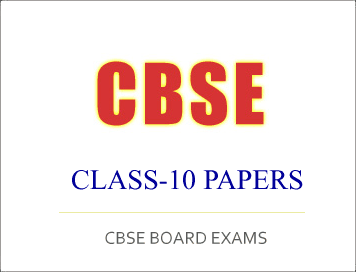Disclaimer: This website is not at associated with CBSE, For official website of CBSE visit - www.cbse.nic.in

##SAMPLE QUESTION PAPER (Set - II)
Summative Assessment – II
Class-X (2015–16)
Mathematics

Time: 3 hours

M. M. : 90

General Instructions:
1. All questions are compulsory.
2. The question paper consists of 31 questions divided into four sections A, B, C and D.
3. Section A contains 4 questions of 1 mark each. Section B contains 6 questions of 2 marks each. Section C contains 10 questions of 3 marks each. Section D contains 11 questions of 4 marks each.
4. Use of calculators is not permitted.

Section A

(Question numbers 1 to 4 carry 1 mark each)
1. Find the value of k, for which one root of the quadratic equation kx2-14x+8=0 is six times the other.
2. Find the tenth term of the sequence √2, √8, √18, ………
3. Out of 200 bulbs in a box, 12 bulbs are defective. One bulb is taken out at random from the box. What is the probability that the drawn bulb is not defective?
4. Find the value of a, for which point P (a/3 , 2) is the midpoint of the line segment joining the points Q (-5, 4) and R(-1, 0).

Section B

(Question numbers 5 to 10 carry 2 marks each)
5. If 2 is a root of the equation x2+kx+12=0 and the equation x2+kx+q=0 has equal roots, find the value of q.